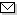Language:   Search:   Contact# Search and Browse the MSC 2000

Search:
Please note that in the second half of 2009, MSC2010 is deployed in the production of ZMATH and will become official standard in January 2010.

Enter a query and click »Search«...

65-XX Numerical analysis → ZMATH
65-00 General reference works (handbooks, dictionaries, bibliographies, etc.) → ZMATH
65-01 Instructional exposition (textbooks, tutorial papers, etc.) → ZMATH
65-02 Research exposition (monographs, survey articles) → ZMATH
65-03 Historical (must also be assigned at least one classification number from Section 01) → ZMATH
65-04 Explicit machine computation and programs (not the theory of computation or programming) → ZMATH
65-05 Experimental papers → ZMATH
65-06 Proceedings, conferences, collections, etc. → ZMATH
65A05 Tables → ZMATH
65Bxx Acceleration of convergence → ZMATH
65Cxx Probabilistic methods, simulation and stochastic differential equations {For theoretical aspects, see 68U20 and 60H35} → ZMATH
65Dxx Numerical approximation and computational geometry {Primarily algorithms; for theory, see 41-XX and 68Uxx} → ZMATH
65E05 Numerical methods in complex analysis (potential theory, etc.) {For numerical methods in conformal mapping, see 30C30} → ZMATH
65Fxx Numerical linear algebra → ZMATH
65Gxx Error analysis and interval analysis → ZMATH
65Hxx Nonlinear algebraic or transcendental equations → ZMATH
65Jxx Numerical analysis in abstract spaces → ZMATH
65Kxx Mathematical programming, optimization and variational techniques → ZMATH
65Lxx Ordinary differential equations → ZMATH
65Mxx Partial differential equations, initial value and time-dependent initial-boundary value problems → ZMATH
65Nxx Partial differential equations, boundary value problems → ZMATHAbel prize 2010I. M. Gelfand 1913-2009MSC2010